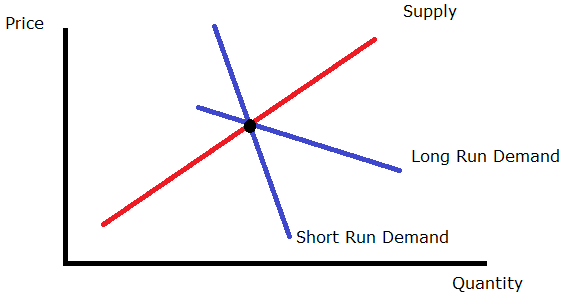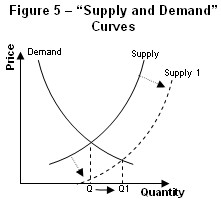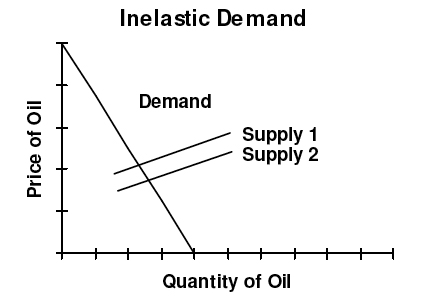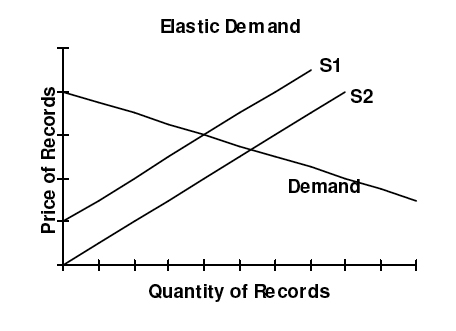# 6.3: Price Elasticity of Supply

## Definition of Price Elasticity of Supply

The price elasticity of supply is the measure of the responsiveness in quantity supplied to a change in price for a specific good.

learning objectives

• Differentiate between the price elasticity of demand for elastic and inelastic goods

In economics, elasticity is a summary measure of how the supply or demand of a particular good is influenced by changes in price. Elasticity is defined as a proportionate change in one variable over the proportionate change in another variable:

$\text{Elasticity}=\dfrac{\% \text{Change in quantity}}{\% \text{Change in price}}$

The price elasticity of supply (PES) is the measure of the responsiveness in quantity supplied (QS) to a change in price for a specific good (% Change QS / % Change in Price). There are numerous factors that directly impact the elasticity of supply for a good including stock, time period, availability of substitutes, and spare capacity. The state of these factors for a particular good will determine if the price elasticity of supply is elastic or inelastic in regards to a change in price.

The price elasticity of supply has a range of values:

• PES > 1: Supply is elastic.
• PES < 1: Supply is inelastic.
• PES = 0: The supply curve is vertical; there is no response of demand to prices. Supply is “perfectly inelastic.”
• PES = ∞∞ (i.e., infinity): The supply curve is horizontal; there is extreme change in demand in response to very small change in prices. Supply is “perfectly elastic.”

Inelastic goods are often described as necessities. A shift in price does not drastically impact consumer demand or the overall supply of the good because it is not something people are able or willing to go without. Examples of inelastic goods would be water, gasoline, housing, and food.

Elastic goods are usually viewed as luxury items. An increase in price for an elastic good has a noticeable impact on consumption. The good is viewed as something that individuals are willing to sacrifice in order to save money. An example of an elastic good is movie tickets, which are viewed as entertainment and not a necessity.

The price elasticity of supply is determined by:

• Number of producers: ease of entry into the market.
• Spare capacity: it is easy to increase production if there is a shift in demand.
• Ease of switching: if production of goods can be varied, supply is more elastic.
• Ease of storage: when goods can be stored easily, the elastic response increases demand.
• Length of production period: quick production responds to a price increase easier.
• Time period of training: when a firm invests in capital the supply is more elastic in its response to price increases.
• Factor mobility: when moving resources into the industry is easier, the supply curve in more elastic.
• Reaction of costs: if costs rise slowly it will stimulate an increase in quantity supplied. If cost rise rapidly the stimulus to production will be choked off quickly.

The result of calculating the elasticity of the supply and demand of a product according to price changes illustrates consumer preferences and needs. The elasticity of a good will be labelled as perfectly elastic, relatively elastic, unit elastic, relatively inelastic, or perfectly inelastic.Price elasticity over time: This graph illustrates how the supply and demand of a product are measured over time to show the price elasticity.Perfectly Inelastic Supply: A graphical representation of perfectly inelastic supply.

## Measuring the Price Elasticity of Supply

The price elasticity of supply is the measure of the responsiveness of the quantity supplied of a particular good to a change in price.

learning objectives

• Calculate elasticities and describe their meaning

The price elasticity of supply (PES) is the measure of the responsiveness of the quantity supplied of a particular good to a change in price (PES = % Change in QS / % Change in Price). The intent of determining the price elasticity of supply is to show how a change in price impacts the amount of a good that is supplied to consumers. The price elasticity of supply is directly related to consumer demand.

### Elasticity

The elasticity of a good provides a measure of how sensitive one variable is to changes in another variable. In this case, the price elasticity of supply determines how sensitive the quantity supplied is to the price of the good.

### Calculating the PES

When calculating the price elasticity of supply, economists determine whether the quantity supplied of a good is elastic or inelastic. The percentage of change in supply is divided by the percentage of change in price. The results are analyzed using the following range of values:

• PES > 1: Supply is elastic.
• PES < 1: Supply is inelastic.
• PES = 0: Supply is perfectly inelastic. There is no change in quantity if prices change.
• PES = infinity: Supply is perfectly elastic. An decrease in prices will lead to zero units produced.

### Factors that Influence the PES

There are numerous factors that impact the price elasticity of supply including the number of producers, spare capacity, ease of switching, ease of storage, length of production period, time period of training, factor mobility, and how costs react.

The price elasticity of supply is calculated and can be graphed on a demand curve to illustrate the relationship between the supply and price of the good.## Applications of Elasticities

In economics, elasticity refers to how the supply and demand of a product changes in relation to a change in the price.

learning objectives

• Give examples of inelastic and elastic supply in the real world

In economics, elasticity refers to the responsiveness of the demand or supply of a product when the price changes.

The technical definition of elasticity is the proportionate change in one variable over the proportionate change in another variable. For example, to determine how a change in the supply or demand of a product is impacted by a change in the price, the following equation is used: Elasticity = % change in supply or demand / % change in price.

The price is a variable that can directly impact the supply and demand of a product. If a change in the price of a product significantly influences the supply and demand, it is considered “elastic.” Likewise, if a change in product price does not significantly change the supply and demand, it is considered “inelastic.”

For elastic demand, when the price of a product increases the demand goes down. When the price decreases the demand goes up. Elastic products are usually luxury items that individuals feel they can do without. An example would be forms of entertainment such as going to the movies or attending a sports event. A change in prices can have a significant impact on consumer trends as well as economic profits. For companies and businesses, an increase in demand will increase profit and revenue, while a decrease in demand will result in lower profit and revenue.

For inelastic demand, the overall supply and demand of a product is not substantially impacted by an increase in price. Products that are usually inelastic consist of necessities like food, water, housing, and gasoline. Whether or not a product is elastic or inelastic is directly related to consumer needs and preferences. If demand is perfectly inelastic, then the same amount of the product will be purchased regardless of the price.

Economists study elasticity and use demand curves in order to diagram and study consumer trends and preferences. An elastic demand curve shows that an increase in the supply or demand of a product is significantly impacted by a change in the price. An inelastic demand curve shows that an increase in the price of a product does not substantially change the supply or demand of the product.Inelastic Demand: For inelastic demand, when there is an outward shift in supply and prices fall, there is no substantial change in the quantity demanded.Elastic Demand: For elastic demand, when there is an outward shift in supply, prices fall which causes a large increase in quantity demanded.

## Key Points

• Elasticity is defined as a proportionate change in one variable over the proportionate change in another variable: $$\text{Elasticity}=\dfrac{\% \text{Change in quantity}}{\% \text{Change in price}}$$
• The impact that a price change has on the elasticity of supply also directly impacts the elasticity of demand.
• Inelastic goods are often described as necessities, while elastic goods are considered luxury items.
• The elasticity of a good will be labelled as perfectly elastic, relatively elastic, unit elastic, relatively inelastic, or perfectly inelastic.
• The price elasticity of supply = % change in quantity supplied / % change in price.
• When calculating the price elasticity of supply, economists determine whether the quantity supplied of a good is elastic or inelastic.
• PES > 1: Supply is elastic. PES < 1: Supply is inelastic. PES = 0: if the supply curve is vertical, and there is no response to prices. PES = infinity: if the supply curve is horizontal.
• To determine the elasticity of a product, the proportionate change of one variable is placed over the proportionate change of another variable (Elasticity = % change of supply or demand / % change in price ).
• For elastic demand, a change in price significantly impacts the supply and demand of the product.
• For inelastic demand, a change in the price does not substantially impact the supply and demand of the product.
• Economists use demand curves in order to document and study elasticity.

## Key Terms

• luxury: Something very pleasant but not really needed in life.
• supply: The amount of some product that producers are willing and able to sell at a given price, all other factors being held constant.
• demand: The desire to purchase goods and services.
• mobility: The ability for economic factors to move between actors or conditions.
• capacity: The maximum that can be produced on a machine or in a facility or group.
• elastic: Sensitive to changes in price.
• demand: The desire to purchase goods and services.
• inelastic: Not sensitive to changes in price.
• supply: The amount of some product that producers are willing and able to sell at a given price, all other factors being held constant.